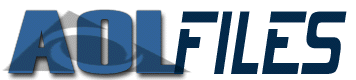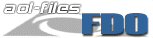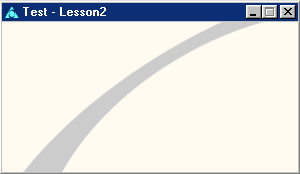Mail:  Username:  Password:   Help | Sign Up Search:FDO Tutorial: Lesson 2 by Tau In this tutorial the basics of designing forms will be taught. First off, every action, including creating forms begins with the atom uni_start_stream. Next comes the properties of a form. Below are few of the properties that a form can have. All of the following atoms are descriped in the atom database. mat_orientation - describes the shape of the objects width relavitve to its height. We are just going to use "vff" for now because there are so many. mat_color_face - sets the background color of the form. mat_bool_background_flood - This must be Yes in order for mat_color_face to take effect. mat_position - controls where the form is when it loads. First part of the argument is the row it is in. It is either top, center, or bottom. This is followed by an underscore (_) then left, center, or right. So if you wanted the form to start in the middle of the screen and at the top you would use top_center. mat_bool_resize_vertical - controls whether the form can be resized. If either the atom mat_bool_resize_horizontal or this is No then the form will not have a maximize button. mat_precise_width <#> - Determines the width of a form measured in pixels. mat_bool_precise must be Yes. mat_precise_height <#> - Determines the height of a form measured in pixels. mat_bool_precise must be Yes. mat_precise_x <#> - Controls where the form starts. If the number is 0 then it will be 0 pixels across from the top left corner of AOL and down however many units specified in mat_precise_y. mat_precise_y <#> - Controls where the form starts. If the number is 0 then it will be 0 pixels down from the top left corner of AOL and acrosss however many units specified in mat_precise_x. mat_bool_precise - Determines whether the form is the exact width and height as specified above. mat_art_id - If mat_bool_background_pic is Yes then the form will have a picture background wit the specified id. mat_bool_background_pic - Determines whether the form has a background image defined by mat_art_id. Every window also has a name. The name for a form is ind_group. The code to start a form is as follows: man_start_object Where caption is the form's caption. Example #1 Now to combine everything that has just been explained. Save the following FDO stream and play it using the methods explained in Lesson 1. ```uni_start_stream man_start_object mat_orientation mat_position mat_bool_resize_vertical mat_precise_width <300> mat_precise_height <150> mat_bool_precise mat_art_id <1-1-40898> mat_bool_background_pic man_end_object man_update_display uni_end_stream ``` Will result in:Example #2 ``` uni_start_stream man_start_object mat_orientation mat_position mat_bool_resize_vertical mat_color_face <0, 102, 153> mat_bool_background_flood mat_precise_width <400> mat_precise_height <100> mat_bool_precise man_end_object man_update_display uni_end_stream ``` Will result in:Next week the focus will be on the objects that can be on a form.
 © 2000 BMB and Tau Productions. Contacts: BMB | Tau | Rob